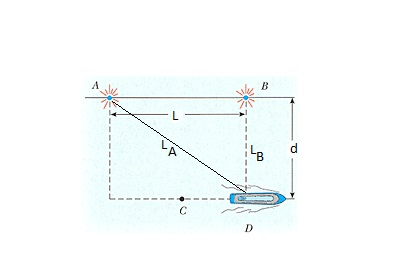# A ship travels along a straight line parallel to the shore and a distance d = 580 m from it. The...

## Question:

A ship travels along a straight line parallel to the shore and a distance d = 580 m from it. The ship's radio receives simultaneous signals of the same frequency from antennas A and B, separated by a distance L = 700 m apart. The signals interfere constructively at point C, which is equidistant from A and B. The signal goes through the first minimum at point D, which is directly outward from the shore from point B.

Determine the wavelength of the radio waves.

## Interference:

The superposition of the waves is known as interference. If the waves are in phase while superposition, then the interference would be constructive, and if the waves are out of the phase, then the interference would be destructive.

Therefore, for the destructive interference, the path difference between the waves must be equal to the half of the wavelength.Given Data:

• Distance between the antennas{eq}\rm (L) = 700 \ m {/eq}
• Distance of the ship from the shore {eq}\rm (d) = 580 \ m {/eq}

The path length of the wave generating from Anteena B, would be

{eq}\rm L_{B} = d \\ L_{B} = 580 \ m {/eq}

The path length of the wave generating from Anteena A

{eq}\rm L_{A} = \sqrt{d^{2} + L^{2}} \\ L_{A} = \sqrt{580^{2} + 700^{2}} \\ L_{A} = 909 \ m {/eq}

The condition for the destructive interference is given by

{eq}\begin{align} \rm L_{A} - L_{B} &= \rm \dfrac{\lambda}{2} \\ 909- 580 &= \dfrac{\lambda}{2} \\ \lambda &= \rm 165 \ m \\ \end{align} {/eq}